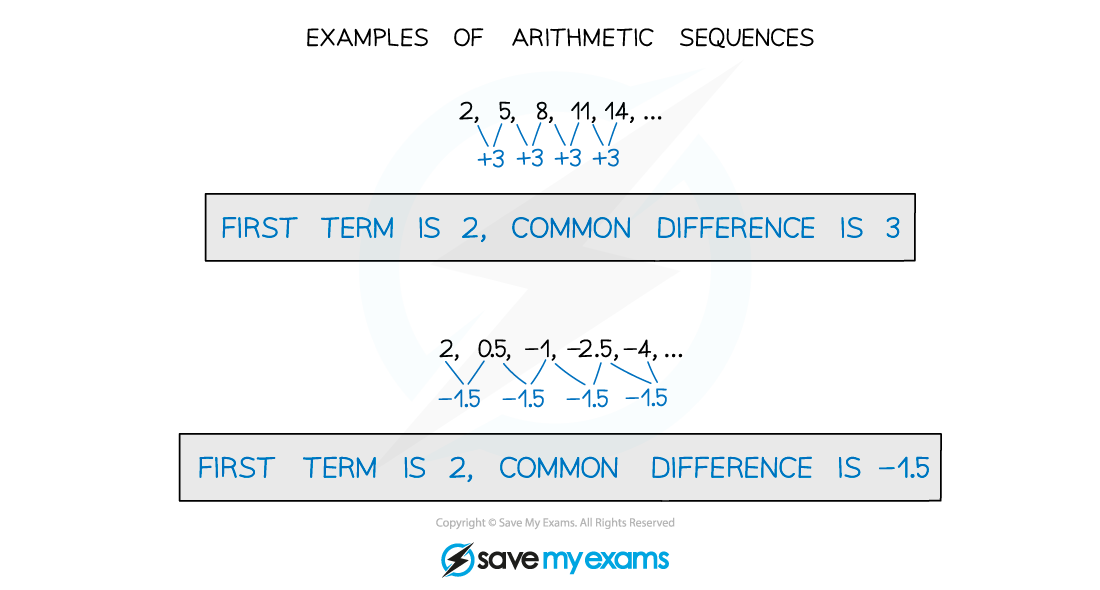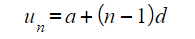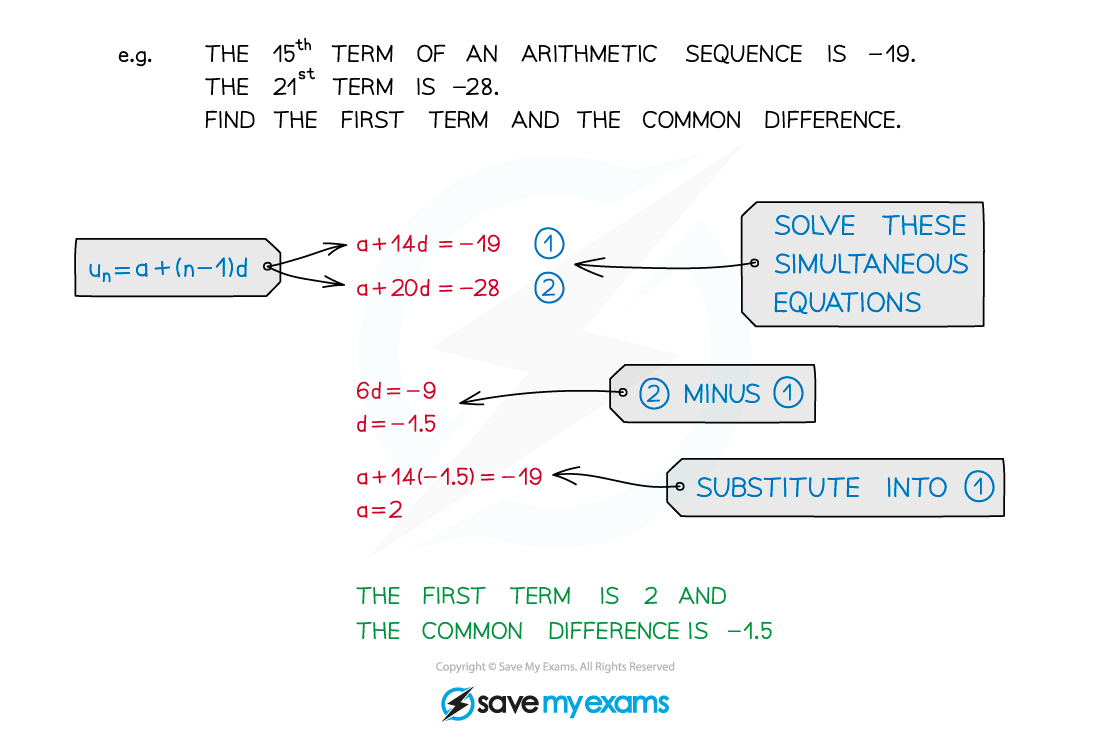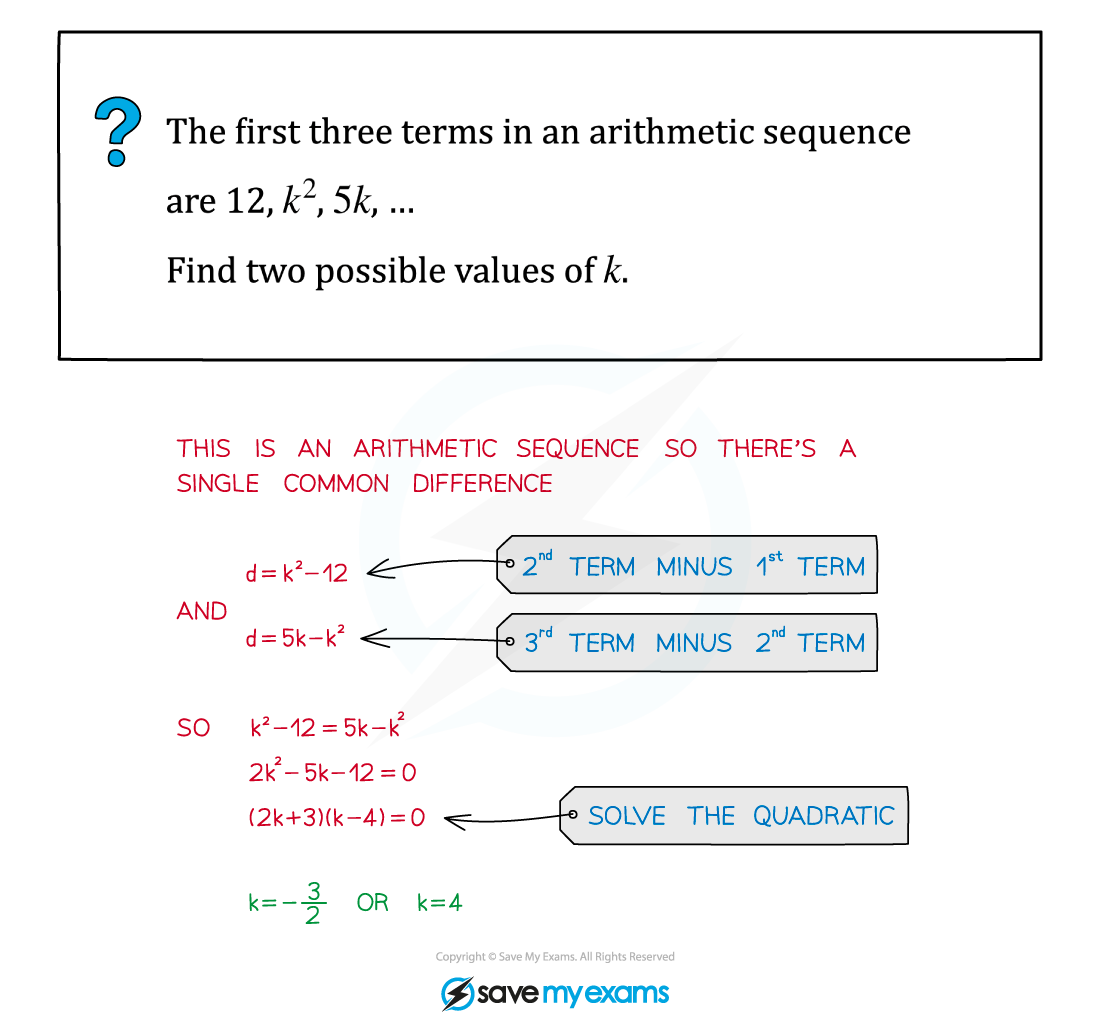# AQA A Level Maths: Pure复习笔记4.3.1 Arithmetic Sequences

### Arithmetic Sequences

#### What do I need to know about arithmetic sequences?

• In an arithmetic sequence, the difference between consecutive terms in the sequence is constant• That constant difference is known as the common difference of the sequence
• You need to know the nth term formula for an arithmetic sequence
•• a is the first term
• d is the common difference

#### Exam Tip

If you know two terms in an arithmetic sequence you can find a and d using simultaneous equations.#### Worked Example